##All rectangular hyperbolas inscribed in a triangle

Problem: Construct all rectangular hyperbolas inscribed in a triangle (so that all sides of the triangle are tangent to the hyperbola).
If the triangle is to be tangent to a rectangular hyperbola, then two of its sides must be tangent to the same branch of the hyperbola. Hence the angle of these sides must be greater than the angle of the asymptotic lines, which is a right angle. Thus only obtuse-angled triangle can have circumscribed hyperbolas.
The crucial fact is that, if such an hyperbola exists, then the conjugate circle (c) of the triangle passes through the center O of the hyperbola. Besides O can be any point of the conjugate circle of the triangle.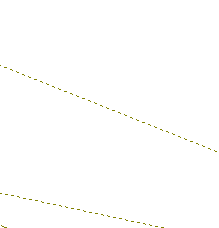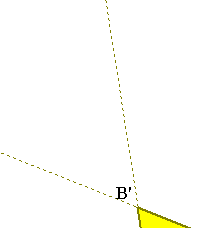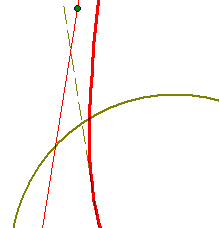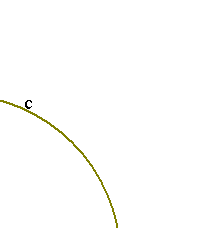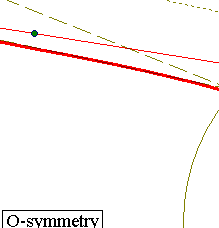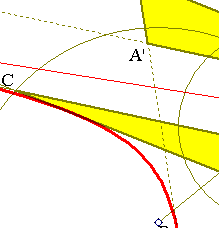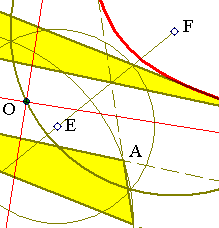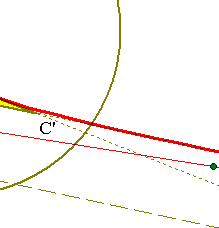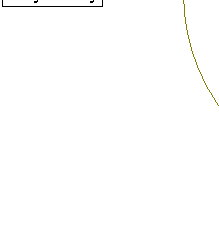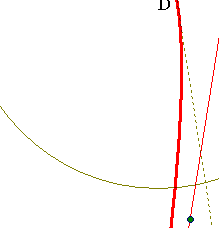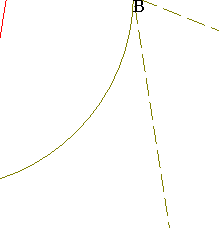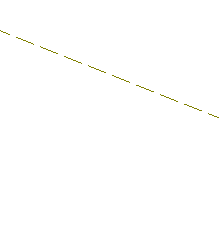The previous remarks give a recipe for the actual construction of all rectangular hyperbolas inscribed in an obtuse-angled triangle ABC. In fact, consider an arbitrary point O of the conjugate circle (c) of the triangle and take the symmetric A'B'C' of the triangle w.r. to O. The hyperbola has to be tangent to the sides of ABC as well as the sides of A'B'C'. Hence it can be constructed as the conic simultaneously tangent to 5 out of the six sides of ABC and A'B'C'.
The above picture shows the circumcircle of ABC with center D, its Euler circle with center E and its conjugate circle c with center F, symmetric of D w.r. to E.
For an analytic proof of the crucial fact referred above see: Aubert, P & Papelier, G. Exercices de Geometrie Analytique Paris, Librairie Vuibert, 1966, p. 252.

FourEulerCircles.html
Hyperbola.html
HyperbolaAsymptoticProperty.html
HyperbolaAsymptotics.html
HyperbolaRectangular.html
NinePointsConic.html
OrthoRectangular.html
RectangularAsProp.html
RectHypeCircumscribed.html
RectHyperbolasTangent4Lines.html
RectHypeThroughFourPts.html